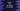# Different ways in Python to find the square of a number## Python program to find the square of a number :

We have different ways to find the square of a number in python. In this post, I will show you different ways to do that with examples.

## Example 1 : Using arithmetic operator :

Arithmetic operator * is used to multiply two numbers. The below program will take one number as input from the user, calculates the square and prints it out :

``````num = int(input("Enter a number : "))

num_square = num * num

print("Square of {} is {}".format(num, num_square))``````

Here, it asks the user to enter a number, reads it and stores it in variable num. Then, it calculates the square value for that number and store it in numsquare_.

Sample output :

``````Enter a number : 3
Square of 3 is 9

Enter a number : 4
Square of 4 is 16``````

## Example 2 : Using exponent operator :

Exponent operator ** can be used to find the square of a number. 4**3 means 4 being raised to the 3rd power. Similarly, 4**2 is the square of 4. So, we can read the user input number and raise it to second power using this operator.

``````num = int(input("Enter a number : "))

num_square = num ** 2

print("Square of {} is {}".format(num, num_square))``````

#### Sample output :

``````Enter a number : 4
Square of 4 is 16

Enter a number : 5
Square of 5 is 25

Enter a number : 11
Square of 11 is 121``````

## Example 3: Using math.pow() :

Python math library provides one method called pow() to calculate the power of a number. It takes two parameters. pow(x,y) means x to the power y. If we use y as 2, it will give us the square of a number.

``````import math

num = int(input("Enter a number : "))

num_square = math.pow(num, 2)

print("Square of {} is {}".format(num, num_square))``````

Sample output :

``````Enter a number : 12
Square of 12 is 144.0

Enter a number : 4
Square of 4 is 16.0

Enter a number : 3
Square of 3 is 9.0``````